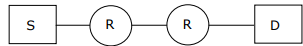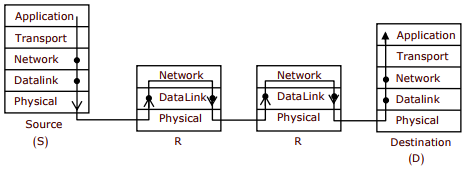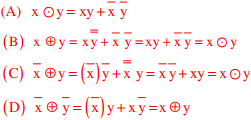# CS GATE 2013 - Online Test

Q1. Choose the grammatically INCORRECT sentence:
Answer : Option C
Explaination / Solution:
No Explaination.

Q2. Assume that source S and destination D are connected through two intermediate routers labeled R. Determine how many times each packet has to visit the network layer and the data link layer during a transmission from S to D.Answer : Option C
Explaination / Solution:From above given diagram, its early visible that packet will visit network layer 4 times, once at each node [S, R, R, D] and packet will visit Data Link layer 6 times. One time at S and one time at D, then two times for each intermediate router R as data link layer is used for link to link communication.
Once at packet reaches R and goes up from physical –DL-Network and second time when packet coming out of router in order Network – DL- Physical

Q3. After several defeats in wars, Robert Bruce went in exile and wanted to commit suicide. Just before committing suicide, he came across a spider attempting tirelessly to have its net. Time and again, the spider failed but that did not deter it to refrain from making attempts. Such attempts by the spider made Bruce curious. Thus, Bruce started observing the nearimpossible goal of the spider to have the net. Ultimately, the spider succeeded in having its net despite several failures. Such act of the spider encouraged Bruce not to commit suicide. And then, Bruce went back again and won many a battle, and the rest is history. Which one of the following assertions is best supported by the above information?
Answer : Option D
Explaination / Solution:
No Explaination.

Q4. The transport layer protocols used for real time multimedia, file transfer, DNS and email, respectively are
Answer : Option C
Explaination / Solution:

Real time multimedia needs connectionless service, so under lying transport layer protocol used is UDP File transfer rums over TCP protocol with port no-21 DNS runs over UDP protocol within port no-53 Email needs SMTP protocol which runs over TCP protocol within port no – 25

Q5.  Match the problem domains in Group I with the solution technologies in Group II
Group I                                                                     Group II
(p) Services oriented computing                                      (1) Interoperability
(q) Heterogeneous communicating systems                    (2) BPMN
(R) Information representation                                         (3) Publish-find bind
(S) Process description                                                    (4) XML
Answer : Option C
Explaination / Solution:
No Explaination.

Q6. In a k-way set associative cache, the cache is divided into v sets, each of which consists of k lines. The lines of a set are placed in sequence one after another. The lines in set s are sequenced before the lines in set (s+1). The main memory blocks are numbered 0 onwards. The main memory block numbered j must be mapped to any one of the cache lines from
Answer : Option A
Explaination / Solution:

Position of main memory block in the cache (set) = (main memory block number) MOD (number of sets in the cache). As the lines in the set are placed in sequence, we can have the lines from 0 to (K – 1) in each set. Number of sets = v, main memory block number = j First line of cache = (j mod v)*k; last line of cache = (j mod v)*k + (k – 1)

Q7. In an IPv4 datagram, the M bit is 0, the value of HLEN is 10, the value of total length is 400 and the fragment offset value is 300. The position of the datagram, the sequence numbers of the first and the last bytes of the payload, respectively are
Answer : Option C
Explaination / Solution:

M= 0 – Means there is no fragment after this, i.e. Last fragment
HLEN=10 - So header length is 4×10=40, as 4 is constant scale factor
Total Length = 400(40 Byte Header + 360 Byte Payload)
Fragment Offset = 300, that means 300×8 Byte = 2400 bytes are before this last fragment
So the position of datagram is last fragment
Sequence number of First Byte of Payload = 2400 (as 0 to 2399 Sequence no are used)
Sequence number of Last Byte of Payload = 2400+360-1=2759

Q8. Which one of the following expressions does NOT represent exclusive NOR of x and y?
Answer : Option D
Explaination / Solution:Q9. What is the time complexity of Bellman-Ford single-source shortest path algorithm on a complete graph of n vertices?
Answer : Option C
Explaination / Solution:

Bellman-ford time complexity: Θ(|V| × |E|)
For complete graph:  |E| = n(n - 1)/2
|V| = n
Θ(n × (n(n - 1)/2) = Θ(n3)

Q10. The smallest integer than can be represented by an 8-bit number in 2’s complement form is
Answer : Option B
Explaination / Solution: